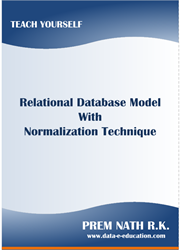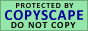Armstrong's Axioms Rules

FD1 - Reflexivity Rule

If Y Í X, then X ® Y

FD2 - Augmentation Rule

If Z Í W and X ® Y, then XW ® YZ

FD3 - Transitivity Rule

If X ® Y and Y ® Z, then X ® Z

There are three additional rules that are implied by the above rules.

FD4 - Pseudotransitivity Rule

If X ® Y and YW ® Z, then XW ® Z

FD5 - Union Rule

If X ® Y and X ® Z, then X ® YZ

FD6 - Decomposition Rule

If X ® YZ, then X ® Y and X ® Z

The rules of union and decomposition imply that we can always replace a set of functional dependencies with an equivalent set that has only functional dependencies of the form X ® A (i.e., the right-hand side contains a single attribute).Your Comment To AUTHORDownload e-Book